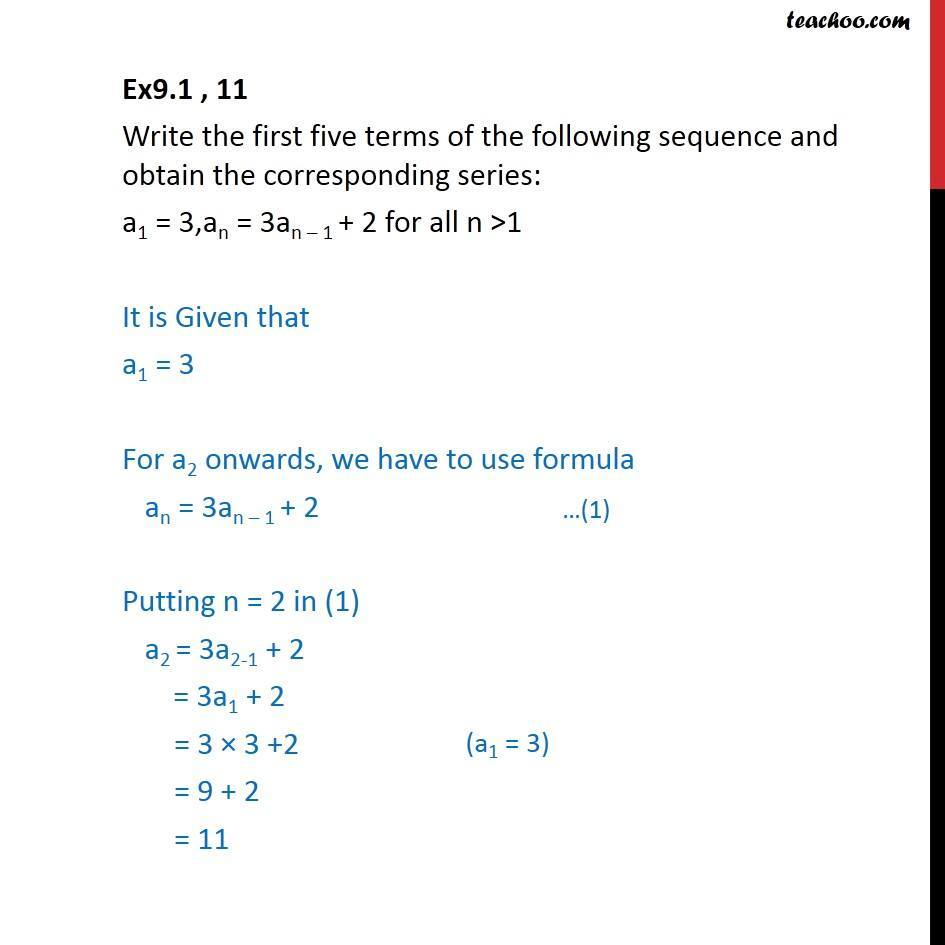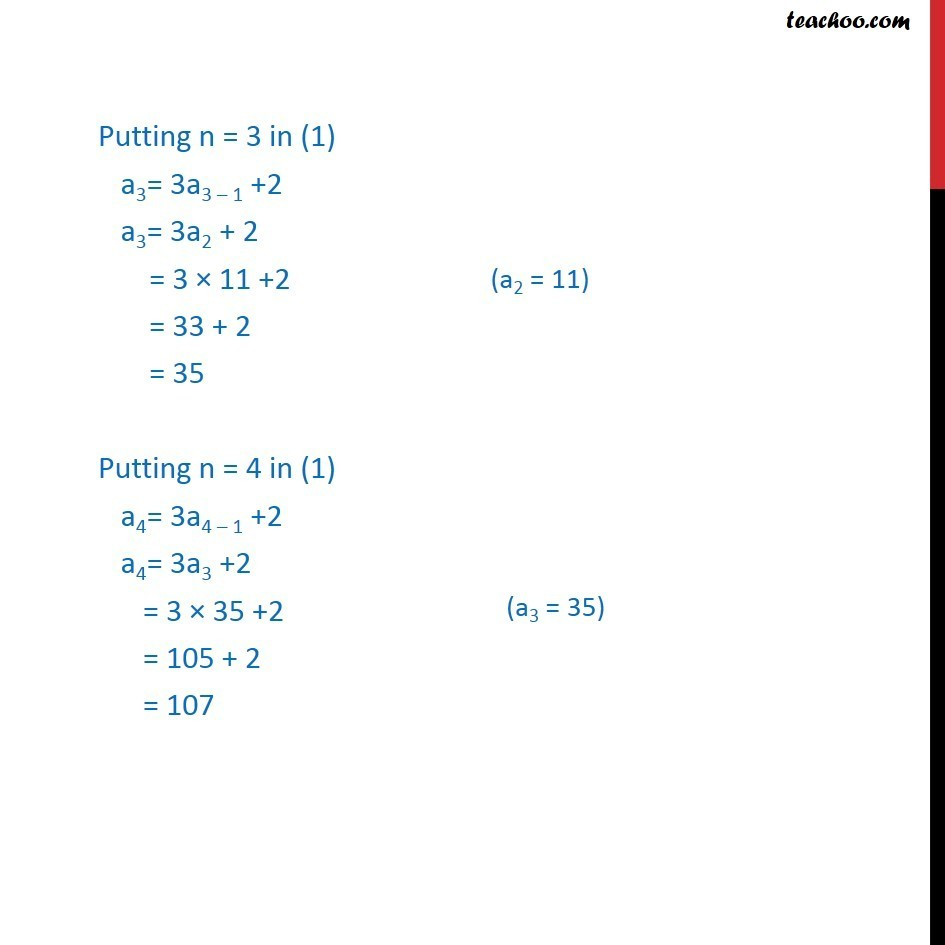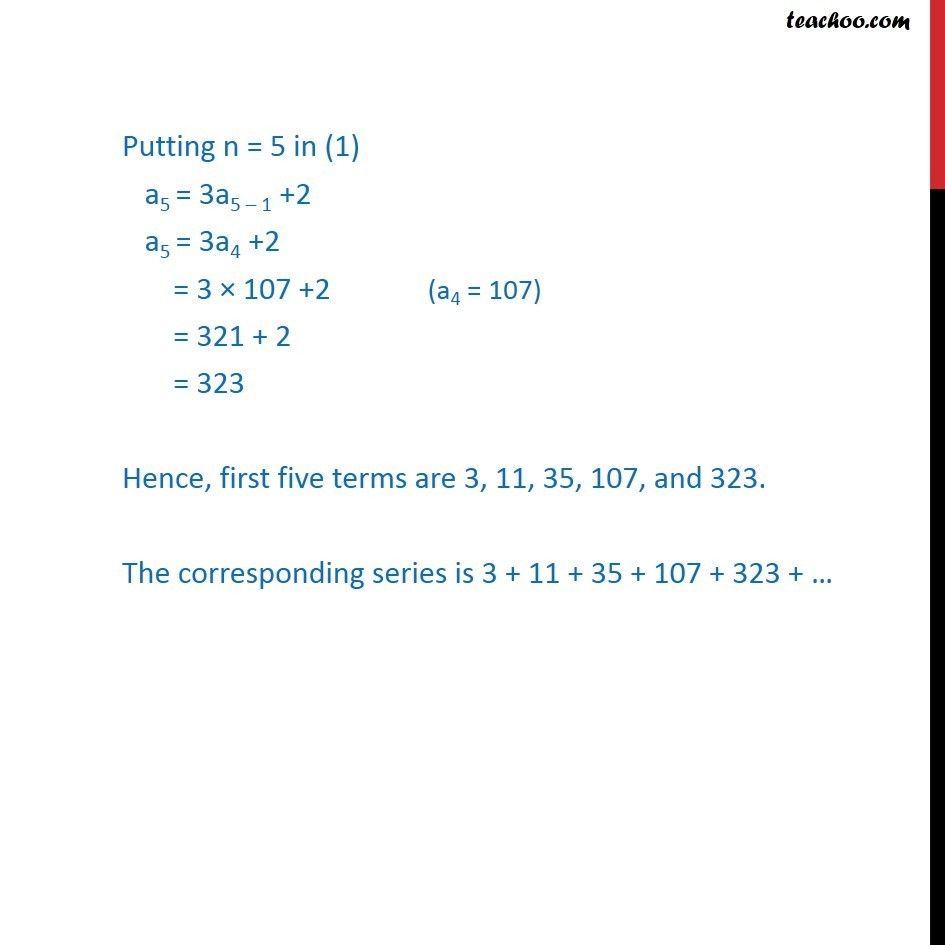Ex 8.1

Chapter 8 Class 11 Sequences and Series
Serial order wiseLearn in your speed, with individual attention - Teachoo Maths 1-on-1 Class

### Transcript

Ex9.1 , 11 Write the first five terms of the following sequence and obtain the corresponding series: a1 = 3,an = 3an – 1 + 2 for all n >1 It is Given that a1 = 3 For a2 onwards, we have to use formula an = 3an – 1 + 2 Putting n = 2 in (1) a2 = 3a2-1 + 2 = 3a1 + 2 = 3 × 3 +2 = 9 + 2 = 11 Putting n = 3 in (1) a3= 3a3 – 1 +2 a3= 3a2 + 2 = 3 × 11 +2 = 33 + 2 = 35 Putting n = 4 in (1) a4= 3a4 – 1 +2 a4= 3a3 +2 = 3 × 35 +2 = 105 + 2 = 107 Putting n = 5 in (1) a5 = 3a5 – 1 +2 a5 = 3a4 +2 = 3 × 107 +2 = 321 + 2 = 323 Hence, first five terms are 3, 11, 35, 107, and 323. The corresponding series is 3 + 11 + 35 + 107 + 323 + …